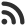Feed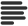Articles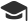Tutorials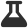CodingLab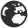CommunityLeaderboardFounder & Programmer at HackersFriend Updated July 12, 2019, 3:32 p.m. ⋅ 125 views

# Remove an element to maximize the GCD of a given array

Problem

You are given an array, let's call it arr [] of lenght N >= 2.  Your task to remove any one element from the array, so that, GCD of whole array is maximized.

Sample Example

Input

``12 15 18``

Output

``6``

Explanation

Here we have 12, 15 and 18 as elements of array, from input. We have to remove any element from array to maximize its GCD.

GCD(15,18) = 3

Now, Let's remove 15.

GCD(12,18) = 6

Let's check by removing 18.

GCD(12,15) = 3

Here, we clearly see GCD(12,18) = 6 is the maximum we can obtain.

Solution

Geneal idea

Since we have to remove only 1 element, what we can do is, we'll generate all possible sub-sequences of size N-1 of this array and find their GCD, after that, the maximum value of GCD would be our answer.

We can do that for sure. But this is very inefficient, once the size of array gets big, this apporach will be very slow.

Optimal approach to find GCD of sub-sequences

We'll use single state dynamic programming.

we'll create 2 arrays, prefixGCD [ ] and sufficGCD [ ].

Then GCD(prefixGCD[i – 1], suffixGCD[i + 1]) will be our required answer.

Let's code this idea.

``````#include <bits/stdc++.h>
using namespace std;

int MaxGCD(int a[], int n)
{

// returns max GCD after removing an element

// Prefix and Suffix arrays
int Prefix[n + 2];
int Suffix[n + 2];

// Single state dynamic programming relation to store gcd of first i elements
// from left in Prefix[i]

Prefix = a;
for (int i = 2; i <= n; i += 1) {
Prefix[i] = __gcd(Prefix[i - 1], a[i - 1]);
}

// Initializing Suffix array
Suffix[n] = a[n - 1];

// Single state dynamic programming relation
// for storing gcd of all the elements having
// greater than or equal to i in Suffix[i]
for (int i = n - 1; i >= 1; i -= 1) {
Suffix[i] = __gcd(Suffix[i + 1], a[i - 1]);
}

// If first or last element of
// the array has to be removed
int ans = max(Suffix, Prefix[n - 1]);

// If any other element is replaced
for (int i = 2; i < n; i += 1) {
ans = max(ans, __gcd(Prefix[i - 1], Suffix[i + 1]));
}

// Return the maximized gcd
return ans;
}

int main()
{
int a;
int n;

// get the total number of elements
cin>>n;

// get input to all elements
for(int i=0; i<n; i++)
{
cin>>a[i];
}

cout << MaxGCD(a, n);

return 0;
}
``````

 arrow_upward UpvoteComment arrow_downward downvote

Events

Nov. 28, 2018, 5:30 p.m.

Python from zero to hero

place Delhi

Aug. 13, 2018, 5:30 p.m.

Python from zero to hero

place Bangalore ( HackersFriend office BTM Layout)